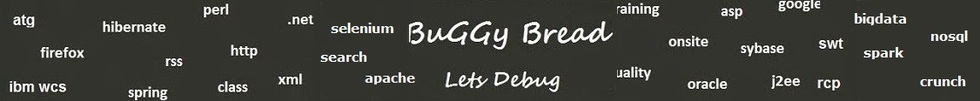## Search Java Classes and Packages

Search Java Frameworks and Libraries

255581 classes and counting ...
 Search Tips Index Status

#Org.jgrapht.alg Classes and Interfaces - 42 results found.
 Name Description Type Package Framework BellmanFordShortestPath algorithm: weights could be negative, paths could be constrained by a maximum number of edges. Class org.jgrapht.alg JGraph BiconnectivityInspector Inspects a graph for the biconnectivity property. Class org.jgrapht.alg JGraph BlockCutpointGraph Definition of a block of a graph in MathWorld. Class org.jgrapht.alg JGraph BronKerboschCliqueFinder This class implements Bron-Kerbosch clique detection algorithm as it is described in [Samudrala R. Class org.jgrapht.alg JGraph ChromaticNumber chromatic number of a graph to be calculated. Class org.jgrapht.alg JGraph CliqueMinimalSeparatorDecomposition Clique Minimal Separator Decomposition using MCS-M+ and Atoms algorithm as described in Berry et al. Class org.jgrapht.alg JGraph ConnectivityInspector Allows obtaining various connectivity aspects of a graph. Class org.jgrapht.alg JGraph CycleDetector Performs cycle detection on a graph. Class org.jgrapht.alg JGraph DijkstraShortestPath An implementation of Dijkstra's shortest path algorithm using ClosestFirstIterator. Class org.jgrapht.alg JGraph DirectedNeighborIndex Maintains a cache of each vertex's neighbors. Class org.jgrapht.alg JGraph DirectedSimpleCycles A common interface for classes implementing algorithms for enumeration of the simple cycles of a directed graph. Interface org.jgrapht.alg.cycle JGraph EdmondsBlossomShrinking An implementation of Edmonds Blossom Shrinking algorithm for constructing maximum matchings on graphs. Class org.jgrapht.alg JGraph EdmondsKarpMaximumFlow directed graph where each edge has a capacity and each edge receives a flow. Class org.jgrapht.alg JGraph EulerianCircuit This algorithm will check whether a graph is Eulerian (hence it contains an circuit). Class org.jgrapht.alg JGraph FloydWarshallShortestPaths Floyd-Warshall algorithm finds all shortest paths (all n^2 of them) in O(n^3) time. Class org.jgrapht.alg JGraph HamiltonianCycle This class will deal with finding the optimal or approximately optimal minimum tour (hamiltonian cycle) or commonly known as the Traveling Class org.jgrapht.alg JGraph HopcroftKarpBipartiteMatching This class is an implementation of the Hopcroft-Karp algorithm which finds a maximum matching in an undirected simple bipartite graph. Class org.jgrapht.alg JGraph JohnsonSimpleCycles Find all simple cycles of a directed graph using the Johnson's algorithm. Class org.jgrapht.alg.cycle JGraph KruskalMinimumSpanningTree An implementation of Kruskal's minimum spanning tree algorithm. Class org.jgrapht.alg JGraph KShortestPaths The algorithm determines the k shortest simple paths in increasing order of weight. Class org.jgrapht.alg JGraph KuhnMunkresMinimalWeightBipartitePerfectMatching Kuhn-Munkres algorithm (named in honor of Harold Kuhn and James Munkres) solving assignment problem also known as hungarian algorithm Class org.jgrapht.alg JGraph KuhnMunkresMinimalWeightBipartitePerfectMatching .KuhnMunkresMatrixImplementation Class org.jgrapht.alg.KuhnMunkresMinimalWeightBipartitePerfectMatching JGraph MatchingAlgorithm Allows to derive matching fromReturns set of edges making up the matching Interface org.jgrapht.alg.interfaces JGraph MinimumSpanningTree minimum spanning tree from given undirected connected graph. Interface org.jgrapht.alg.interfaces JGraph MinSourceSinkCut Given a directed, weighted graph G(V,E). Class org.jgrapht.alg JGraph NaiveLcaFinder Class org.jgrapht.alg JGraph NeighborIndex Maintains a cache of each vertex's neighbors. Class org.jgrapht.alg JGraph PatonCycleBase Find a cycle base of an undirected graph using the Paton's algorithm. Class org.jgrapht.alg.cycle JGraph PrimMinimumSpanningTree An implementation of Prim's algorithm that finds a minimum spanning tree/forest subject to Class org.jgrapht.alg JGraph StoerWagnerMinimumCut Wagner minimum cut algorithm. Class org.jgrapht.alg JGraph StrongConnectivityInspector Complements the ConnectivityInspector class with the capability to compute the strongly connected components of a directed Class org.jgrapht.alg JGraph SzwarcfiterLauerSimpleCycles Find all simple cycles of a directed graph using the Schwarcfiter and Lauer's J. Class org.jgrapht.alg.cycle JGraph TarjanLowestCommonAncestor Class org.jgrapht.alg JGraph TarjanLowestCommonAncestor .LcaRequestResponse Class org.jgrapht.alg.TarjanLowestCommonAncestor JGraph TarjanSimpleCycles Find all simple cycles of a directed graph using the Tarjan's algorithm. Class org.jgrapht.alg.cycle JGraph TiernanSimpleCycles Find all simple cycles of a directed graph using the Tiernan's algorithm. Class org.jgrapht.alg.cycle JGraph TransitiveClosure Constructs the transitive closure of the input graph. Class org.jgrapht.alg JGraph UndirectedCycleBase A common interface for classes implementing algorithms for finding a cycle base of an undirected graph. Interface org.jgrapht.alg.cycle JGraph UnionFind An implementation of Union Find data structure. Class org.jgrapht.alg.util JGraph VertexCovers Algorithms to find a vertex cover for a graph. Class org.jgrapht.alg JGraph VertexDegreeComparator Compares two vertices based on their degree. Class org.jgrapht.alg.util JGraph WeightedMatchingAlgorithm Allows to derive weighted matching from general graphSee Also:MatchingAlgorithm Interface org.jgrapht.alg.interfaces JGraph